# combination of capacitors

## Capacitors in series and parallel combinations

For practical applications , two or more capacitors are often used in combination and their total capacitance C must be known.To find total capacitance of the arrangement of capacitor we would use equation
Q=CV
(i) Parallel combination of capacitors
• Figure below shows two capacitors connected in parallel between two points A and B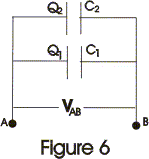• Right hand side plate of capacitors would be at same common potential VA. Similarly left hand side plates of capacitors would also be at same common potential VB.
• Thus in this case potential difference VAB=VA-VB would be same for both the capacitors, and charges Q1 and Q2 on both the capacitors are not necessarily equal. So,
Q1=C1V and Q2=C2V
• Thus charge stored is divided amongst both the capacitors in direct proportion to their capacitance.
• Total charge on both the capacitors is,
Q=Q1+Q2
=V(C1+C2)

and
Q/V=C1+C2                              (8)
So system is equivalent to a single capacitor of capacitance
C=Q/V
where,
• When capacitors are connected in parallel their resultant capacitance C is the sum of their individual capacitances.
• The value of equivalent capacitance of system is greater then the greatest individual one.
• If there are number of capacitors connected in parallel then their equivalent capacitance would be
C=C1+C2+ C3...........               (10)

(ii) Series combination of capacitors
• Figure 7 below shows two capacitors connected in series combination between points A and B.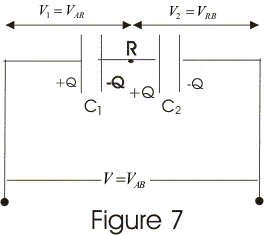• Both the points A and B are maintained at constant potential difference VAB.
• In series combination of capacitors right hand plate of first capacitor is connected to left hand plate of next capacitor and combination may be extended foe any number of capacitors.
• In series combination of capacitors all the capacitors would have same charge.
• Now potential difference across individual capacitors are given by
VAR=Q/C1
and,
VRB=Q/C2
• Sum of VAR and VRB would be equal to applied potential difference V so,
V=VAB=VAR+VRB
=Q(1/C1 + 1/C2)

or,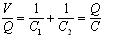where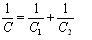i.e., resultant capacitance of series combination C=Q/V, is the ratio of charge to total potential difference across the two capacitors connected in series.
• So, from equation 12 we say that to find resultant capacitance of capacitors connected in series, we need to add reciprocals of their individual capacitances and C is always less then the smallest individual capacitance.
• Result in equation 12 can be summarized for any number of capacitors i.e.,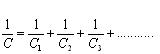Question 1
A network of five capacitors of C is connected to a 100 V supply, as shown in below figure. Determine
(a) the equivalent capacitance of the network
(b) the charge on each capacitor.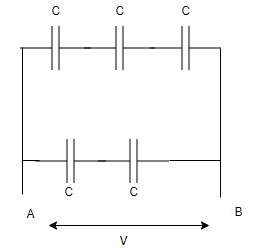Solution
In the given network , the top three Capacitance is in series, So equivalent capacitance of top part
$\frac {1}{C_1} = \frac {1}{C}+\frac {1}{C}+ \frac {1}{C}$
$C_1 = \frac {C}{3}$
Similarly, the lower two Capacitance is in series, So equivalent capacitance of lower part
$\frac {1}{C_2} = \frac {1}{C}+\frac {1}{C}$
$C_2 = \frac {C}{2}$
Now both $C_1$ and $C_2$ are in parallel, so equivalent Capacitance of the Network
$C_{eq} = C_1 + C_2 = \frac {C}{3} + \frac {C}{2}= \frac {5C}{3}$
Now Charge on top part will be
$Q_1 = C_1 V = \frac {CV}{3}$
Now Charge on lower part will be
$Q_2 = C_2 V = \frac {CV}{2}$

Question 2
Find the equivalent Capacitance between point P and Q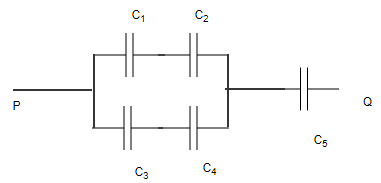Here $C_1=2 \ \mu F$,$C_2=4 \ \mu F$,$C_3=8 \ \mu F$,$C_4=10 \ \mu F$,$C_5=12 \ \mu F$
Solution
In the given network ,$C_1$ and $C_2$ are series, so equivalent capacitance C' for that will be
$\frac {1}{C'}=\frac {1}{C_1}+ \frac {1}{C_2}$
or
$C'=4/3 \ \mu F$
In the given network ,$C_1$ and $C_2$ are series, so equivalent capacitance C'' for that will be
$\frac {1}{C''}=\frac {1}{C_3}+ \frac {1}{C_4}$
or
$C''=40/9 \ \mu F$
Now C' and C'' are in parallel,so it can be replaced with the equivalent capacitance $C_p$
$C_p=C'+ C''=52/9 \mu F$
Now $C_p$ and $C_5$ are in series, so net equivalent capacitance will be
$C_{eq} = \frac { C_p C_5}{C_p + C_5} = \frac {52/9 \times 12}{52/9 + 12} =3.9 \mu F$